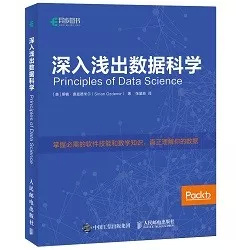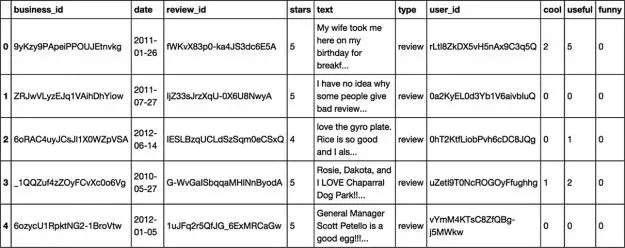# 数据科学入门的5个技巧

Pandas数据格式

• Series
• DataFrame：每个column就是一个Series

统计属性Series： count(),value_counts()，前者是统计总数，后者统计各自value的总数df.isnull() df的空值为True
df.notnull() df的非空值为True

5个步骤概览

• 修改列名

（1）提出有意思的问题；

``````df.rename(columns = {'key':'key2'},inplace=True)
``````

（2）获取数据；

• 更改数据格式astype()

（3）探索数据；

（4）数据建模；

``````isin                 #计算一个“Series各值是否包含传入的值序列中”的布尔数组
unique               #返回唯一值的数组
value_counts         #返回一个Series，其索引为唯一值，值为频率，按计数降序排列
``````

（5）可视化和分享结果。

#### 数据清洗

• 丢弃值drop()
``````df.drop(labels, axis=1)# 按列（axis=1），丢弃指定label的列,默认按行。。。
``````
• 丢弃缺失值dropna()
``````# 默认axi=0（行）；1（列），how=‘any’
df.dropna()#每行只要有空值，就将这行删除
df.dropna(axis=1)#每列只要有空值，整列丢弃
df.dropna(how='all')# 一行中全部为NaN的，才丢弃该行
df.dropna(thresh=3)# 每行至少3个非空值才保留
``````
• 缺失值填充fillna()
``````df.fillna(0)
df.fillna({1:0,2:0.5}) #对第一列nan值赋0，第二列赋值0.5
df.fillna(method='ffill') #在列方向上以前一个值作为值赋给NaN
``````
• 值替换replace()
``````# 将df的A列中 -999 全部替换成空值
df['A'].replace(-999, np.nan)
#-999和1000 均替换成空值
obj.replace([-999,1000],  np.nan)
# -999替换成空值，1000替换成0
obj.replace([-999,1000],  [np.nan, 0])
# 同上，写法不同，更清晰
obj.replace({-999:np.nan, 1000:0})
``````
``````df.duplicated()#两行每列完全一样才算重复，后面重复的为True，第一个和不重复的为false，返回true
#和false组成的Series类型
df.duplicated('key')#两行key这一列一样就算重复

df['A'].unique()# 返回唯一值的数组（类型为array）

df.drop_duplicates(['k1'])# 保留k1列中的唯一值的行，默认保留第一行
df.drop_duplicates(['k1','k2'], take_last=True)# 保留 k1和k2 组合的唯一值的行，take_last=True 保留最后一行
``````

1.1.1　提出有意思的问题

#### 排序

• 索引排序
``````# 默认axis=0，按行索引对行进行排序；ascending=True，升序排序
df.sort_index()
# 按列名对列进行排序，ascending=False 降序
df.sort_index(axis=1, ascending=False)
``````
• 值排序
``````# 按值对Series进行排序，使用order()，默认空值会置于尾部
s = pd.Series([4, 6, np.nan, 2, np.nan])
s.order()

df.sort_values(by=['a','b'])#按列进行排序
``````
• 排名
``````a=Series([7,-5,7,4,2,0,4])
a.rank()#默认method='average'，升序排名（ascending=True），按行（axis=0）
#average 值相等时，取排名的平均值
#min 值相等时，取排名最小值
#max 值相等时，取排名最大值
#first值相等时，按原始数据出现顺序排名
``````

#### 索引设置

• reindex()
更新index或者columns，
默认：更新index，返回一个新的DataFrame
``````# 返回一个新的DataFrame，更新index，原来的index会被替代消失
# 如果dataframe中某个索引值不存在，会自动补上NaN
df2 = df1.reindex(['a','b','c','d','e'])

# fill_valuse为原先不存在的索引补上默认值，不在是NaN
df2 = df1.reindex(['a','b','c','d','e'],  fill_value=0)

# inplace=Ture，在DataFrame上修改数据，而不是返回一个新的DataFrame
df1.reindex(['a','b','c','d','e'],  inplace=Ture)

# reindex不仅可以修改 索引(行)，也可以修改列
states = ["Texas","Utah","California"]
df2 = df1.reindex( columns=states )
``````
• set_index()
将DataFrame中的列columns设置成索引index
打造层次化索引的方法
``````# 将columns中的其中两列：race和sex的值设置索引，race为一级，sex为二级
# inplace=True 在原数据集上修改的

# 默认情况下，设置成索引的列会从DataFrame中移除
# drop=False将其保留下来
``````
• reset_index()
将使用set_index()打造的层次化逆向操作
既是取消层次化索引，将索引变回列，并补上最常规的数字索引
``````df.reset_index()
``````

#### 数据选取

• []
只能对行进 行（row/index） 切片，前闭后开df[0:3]，df[:4]，df[4:]

• where 布尔查找

`````` df[df["A"]>7]
``````
• isin
``````# 返回布尔值
s.isin([1,2,3])
df['A'].isin([1,2,3])
df.loc[df['A'].isin([5.8,5.1])]选取列A中值为5.8，5.1的所有行组成dataframe
``````
• query
多个where整合切片，&：于，|：或
`````` df.query(" A>5.0 & (B>3.5 | C<1.0) ")
``````
• loc ：根据名称Label切片
``````# df.loc[A,B] A是行范围，B是列范围
df.loc[1:4,['petal_length','petal_width']]

# 需求1：创建一个新的变量 test
# 如果sepal_length > 3 test = 1 否则 test = 0
df.loc[df['sepal_length'] > 6, 'test'] = 1
df.loc[df['sepal_length'] <=6, 'test'] = 0

# 需求2：创建一个新变量test2
# 1.petal_length>2 and petal_width>0.3 = 1
# 2.sepeal_length>6 and sepal_width>3 = 2 3.其他 = 0
df['test2'] = 0
df.loc[(df['petal_length']>2)&(df['petal_width']>0.3), 'test2'] = 1
df.loc[(df['sepal_length']>6)&(df['sepal_width']>3), 'test2'] = 2
``````
• iloc：切位置
``````df.iloc[1:4,:]
``````
• ix：混切
名称和位置混切，但效率低，少用
``````df1.ix[0:3,['sepal_length','petal_width']]
``````
• map与lambda
``````alist = [1,2,3,4]
map(lambda s : s+1, alist)#map就是将自定义函数应用于Series每个元素

df['sepal_length'].map(lambda s:s*2+1)[0:3]
``````
• apply和applymap
apply和applymap是对dataframe的操作，前者操作一行或者一列，后者操作每个元素
``````These are techniques to apply function to element, column or dataframe.

Map: It iterates over each element of a series.
df[‘column1’].map(lambda x: 10+x), this will add 10 to each element of column1.
df[‘column2’].map(lambda x: ‘AV’+x), this will concatenate “AV“ at the beginning of each element of column2 (column format is string).

Apply: As the name suggests, applies a function along any axis of the DataFrame.
df[[‘column1’,’column2’]].apply(sum), it will returns the sum of all the values of column1 and column2.
df0[['data1']].apply(lambda s:s+1)

ApplyMap: 对dataframe的每一个元素施加一个函数
func = lambda x: x+2
df.applymap(func), dataframe每个元素加2 (所有列必须数字类型)
``````
• contains
``````# 使用DataFrame模糊筛选数据(类似SQL中的LIKE)
# 使用正则表达式进行模糊匹配,*匹配0或无限次,?匹配0或1次
df_obj[df_obj['套餐'].str.contains(r'.*?语音CDMA.*')]

# 下面两句效果一致
df[df['商品名称'].str.contains("四件套")]
df[df['商品名称'].str.contains(r".*四件套.*")]
``````

1.1.2　获取数据

1.1.3　探索数据

1.1.4　数据建模

1.1.5　可视化和分享结果

1.2.1　数据探索的基本问题

● 数据是有组织格式的，还是无组织格式的？

● 每一行代表什么？

● 每一列代表什么？

● 是否有缺失值？

● 是否需要对某些列进行数据转换？

1.2.2　数据集1：Yelp点评数据

import pandas as pd

● 导入Pandas包，并缩写为pd。

● 读取文件yelp.csv，并命名为yelp_raw_data。

● 查看数据的表头（仅前几行），如图3.1所示。​​图3.1　数据的表头

● 数据源是非常好的行列结构，我们可以认为它是有组织格式的。

● 很明显，每一行代表一条用户的评价。我们还会查看每一行和每一列的数据类型。我们使用DataFrame的shape方法查看数据集的大小，如下所示。

yelp_raw_data.shape

# (10000, 10)

● 结果显示，数据集有10 000行和10列。换言之，数据集有10 000个观测值和10个观测特征。

● date：本列是每条评价的提交日期。请注意，它只精确到了年、月和日。虽然时间通常被认为是连续数据，但本列应该被视为离散数据。本列属于定序尺度，因为日期有天然的顺序。

● review_id: 本列看起来是每条评价的唯一识别码。本列同样属于定类尺度，因为识别码没有天然的顺序。

● stars：本列看起来（别担心，我们随后会对它进行深入的分析）是评价者给每一个餐馆的最终评分。本列是有次序的定性数据，因此属于定序尺度。

● text：本列看起来是用户撰写的评价。对于大部分文本数据，我们将其归为定类尺度。

● type：本列前5行均为“review”，我们猜测它是标记每行是否为“review”的列，也就是说很可能存在不是“review”的行。我们随后将进行更深入的分析。本列属于定类尺度。

● user_id：本列是每个提交评价的用户的唯一识别码。和其他唯一识别码一样，本列也属于定类尺度。

● 使用isnull方法判断是否有缺失值。比如，对于名为awesome_dataframe的DataFrame数据集，使用Python代码awesome_dataframe.isnull().sum()可显示每一列的缺失值总数。

● 我们想知道是否需要改变定量数据的数值范围，或者是否需要为定性数据创建哑变量？由于本数据集只有定性数据，所以我们将焦点放在定序和定类范围。

DataFrame

type(yelp_raw_data)

# pandas.core.frame.Dataframe

DataFrame本质上是一种二维结构，它和电子表格一样以行列结构存储数据。但是相对于电子表格，DataFrame最重要的优点是它可以处理的数据量远超大多数电子表格。

Series

Series是简化版的DataFrame，它只有一个维度。Series本质上是由数据点组成的列表。DataFrame的每一列都可以被看作一个Series对象。下面用代码进行验证。我们首先从DataFrame中抽取单独一列（通常用中括号），代码如下：

yelp_raw_data['business_id']# grab a single column of the Dataframe